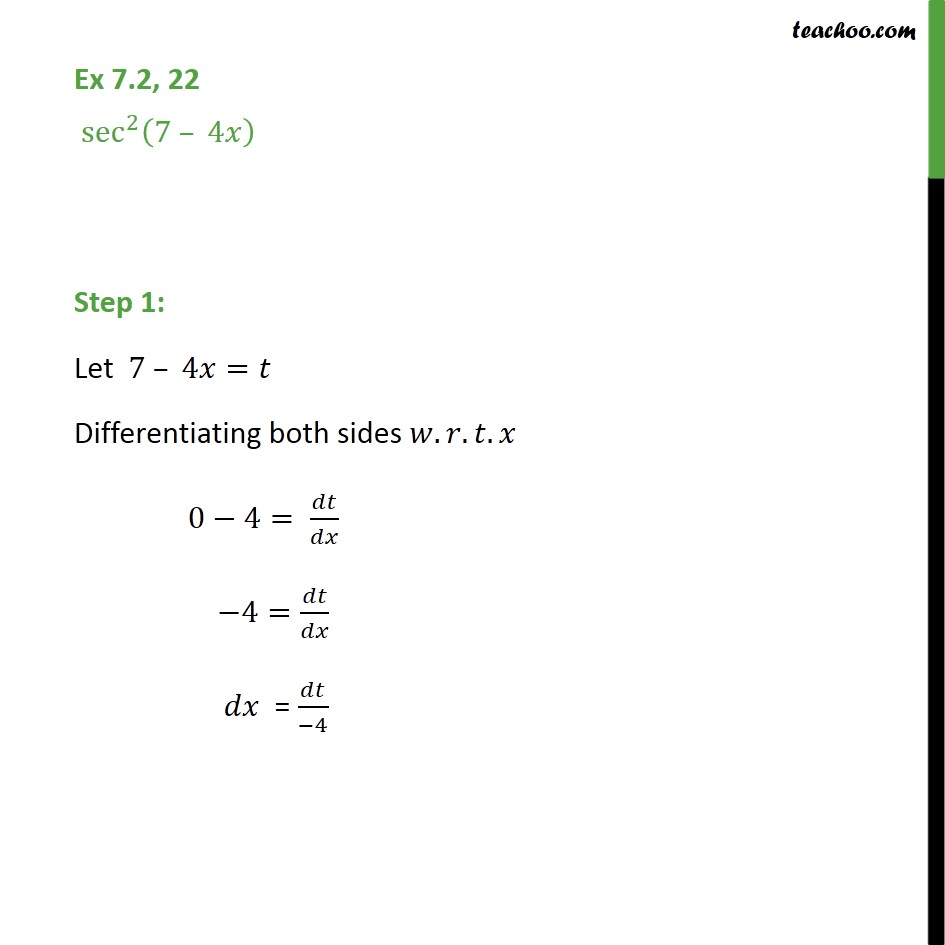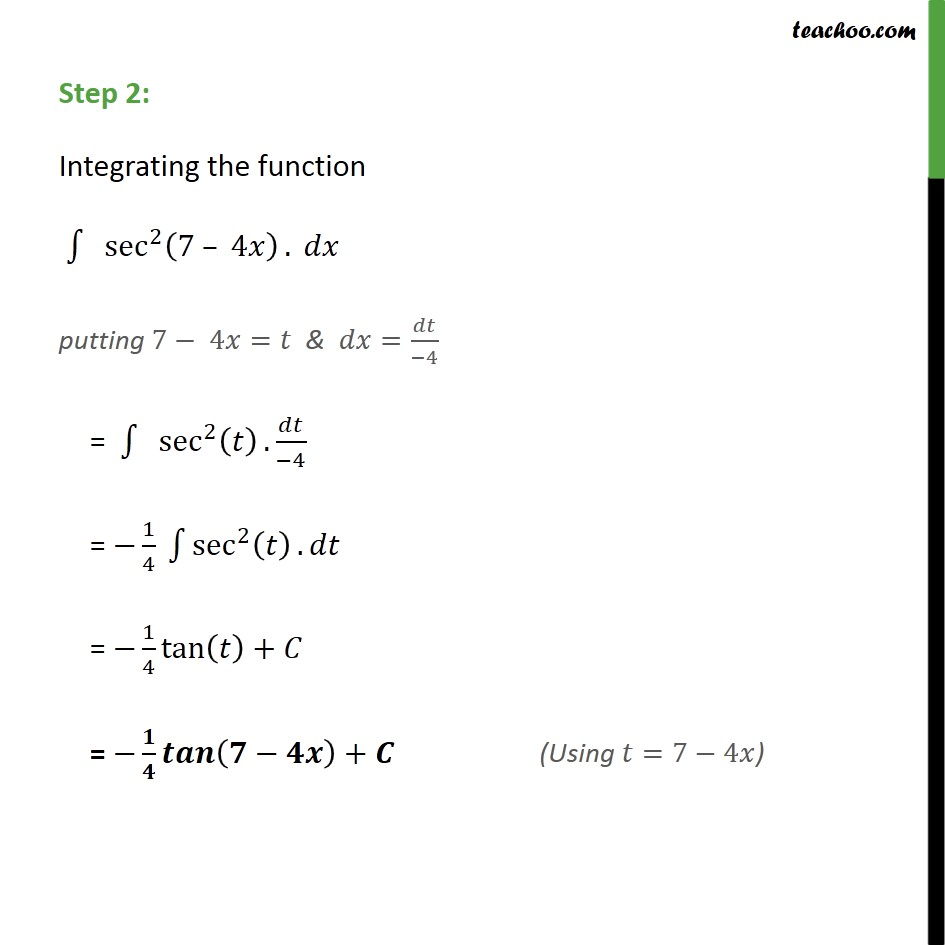1. Chapter 7 Class 12 Integrals
2. Serial order wise
3. Ex 7.2

Transcript

Ex 7.2, 22 sec 2 7 4 Step 1: Let 7 4 = Differentiating both sides . . . 0 4= 4= = 4 Step 2: Integrating the function sec 2 7 4 . putting 7 4 = & = 4 = sec 2 . 4 = 1 4 sec 2 . = 1 4 tan + = +

Ex 7.2

Chapter 7 Class 12 Integrals
Serial order wise